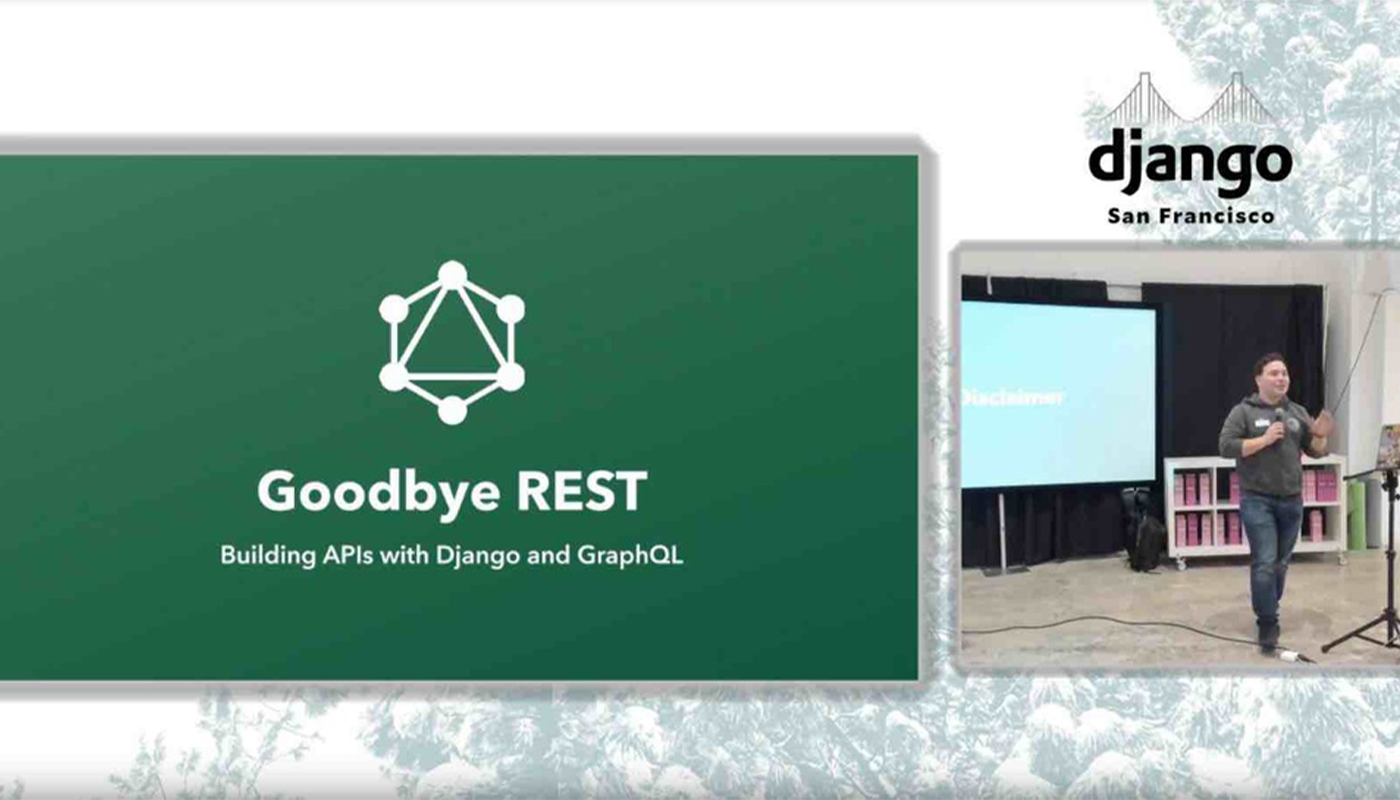Oops! Something went wrong while submitting the form.

# Optimizing JavaScript for runtime speed

By
-
November 3, 2012This summer Brandon Jones of Motorola Mobility presented a talk about efficient JavaScript vector math . The concepts presented in this JavaScript meetup talk are beneficial to make JavaScript run faster in any codebase. Here are some of the principles that a developer should take:

1. Cache variable calls instead of making multiple separate calls. For example,

someObject.innerObject.doSomething();
someObject.innerObject.doSomethingElse();

should be written as:

var a = someObject.innerObject;
a.doSomething();
a.doSomethingElse();

This code is faster because the method lookup on the object only has to be performed once.

2. Write your APIs (application programming interfaces) as function methods not objects.

var theObject = new SomeObject();
theObject.peformOpA();
theObject.peformOpB(paramA, paramB);

should be written as:

var theObject = SomeModule.create(); // create a hashtable of only values
SomeModule.performOpA(theObject);
SomeModule.performOpB(theObject, paramA, paramB);

This code is faster because we are not copying all the function pointers in the object hashtable. Only the values that change between each instance are copied.

3. Inline code rather than writing function calls. Compilers in the early days weren't good at optimizing certain function calls, but they've improved over time now. JavaScript runtime compilers, however, aren't at that level yet.

function pow2(a) {
return a * a;
}
var c = pow2(some_var)

should be written as:

var c = (some_var * some_var);

This code is faster because a function call requires its own execution context (stack frame). Without a function call this extra stack frame isn't needed.

4. Unroll your loops. This concept refers to pipelining instructions without branch conditions. Branch conditions often appear in loops, which prevents the compiler from predicting the code path of execution. Have a look at the example from Brandon (below).

mat3.multiply = function (mat, mat2, dest) {
dest = mat2 * mat + mat2 * mat + mat2 * mat;
dest = mat2 * mat + mat2 * mat + mat2 * mat;
dest = mat2 * mat + mat2 * mat + mat2 * mat;

dest = mat2 * mat + mat2 * mat + mat2 * mat;
dest = mat2 * mat + mat2 * mat + mat2 * mat;
dest = mat2 * mat + mat2 * mat + mat2 * mat;

dest = mat2 * mat + mat2 * mat + mat2 * mat;
dest = mat2 * mat + mat2 * mat + mat2 * mat;
dest = mat2 * mat + mat2 * mat + mat2 * mat;

return dest;
};

This code should be written unrolled.

mat3.multiply = function (mat, mat2, dest) {
var a00 = mat, a01 = mat, a02 = mat,
a10 = mat, a11 = mat, a12 = mat,
a20 = mat, a21 = mat, a22 = mat,

b00 = mat2, b01 = mat2, b02 = mat2,
b10 = mat2, b11 = mat2, b12 = mat2,
b20 = mat2, b21 = mat2, b22 = mat2;

dest = b00 * a00 + b01 * a10 + b02 * a20;
dest = b00 * a01 + b01 * a11 + b02 * a21;
dest = b00 * a02 + b01 * a12 + b02 * a22;

dest = b10 * a00 + b11 * a10 + b12 * a20;
dest = b10 * a01 + b11 * a11 + b12 * a21;
dest = b10 * a02 + b11 * a12 + b12 * a22;

dest = b20 * a00 + b21 * a10 + b22 * a20;
dest = b20 * a01 + b21 * a11 + b22 * a21;
dest = b20 * a02 + b21 * a12 + b22 * a22;

return dest;
};

The code is much faster because bounds checking (i.e., the branch condition) is checked once at the beginning rather than on every call.

5. Use native arrays when performing computation-intensive operations on floating-point numbers. Float32Array is very fast to use but incredibly expensive to create. In other words, reuse and cache floating-point array objects and don't create them inside a loop.

In summary these optimizations are workarounds to JavaScript's non-optimizing compiler. Modern C and Java compilers are able to circumvent some of these issues but web browser JavaScript engines aren't as smart yet. Happy optimizing!## You Might also like...VIDEO: Building API's with Django and GraphQL

At our last Django Meetup Group event, Jayden Windle, the lead engineer at Jetpack, an on demand delivery company, talks building APIs with Django and GraphQL. Watch the video to learn more.Using Pytest to Write Beautiful Tests and a Bulletproof Django App

At the last meeting of the San Francisco Django Meetup Group, Wes Kendall gave a talk on how to make a bulletproof Django application by testing it with pytest. Check out his talk here!Creating a Reusable Component Library: Yeti Lunch and Learn

Part of the Yeti Lunch and Learn series - our amazing developer, Resdan, gives a presentation on creating a reusable component library. Enjoy the video!